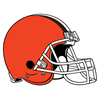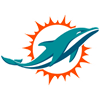DraftKings AFC Championship Odds
+300
3 to 1Bills
21.1% implied probability

+600
6 to 1Chiefs
12.0% implied probability

+750
7.5 to 1Chargers
9.9% implied probability

+850
8.5 to 1Broncos
8.9% implied probability

+1000
10 to 1Browns
7.7% implied probability

+1100
11 to 1Bengals
7.0% implied probability

+1200
12 to 1Ravens
6.5% implied probability

+1200
12 to 1Colts
6.5% implied probability

+1800
18 to 1Titans
4.4% implied probability

+2000
20 to 1Raiders
4.0% implied probability

+2000
20 to 1Dolphins
4.0% implied probability

+2500
25 to 1Patriots
3.2% implied probability

+5000
50 to 1Steelers
1.7% implied probability

+6500
65 to 1Jaguars
1.3% implied probability

+7000
70 to 1Jets
1.2% implied probability

+13000
130 to 1Texans
0.6% implied probability